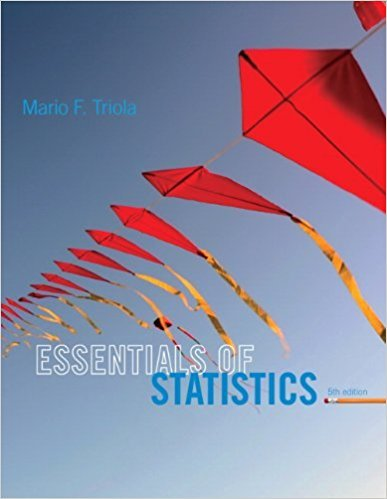×
×

# Solutions for Chapter 9.3: Two Means: Independent Samples## Full solutions for Essentials of Statistics | 5th Edition

ISBN: 9780321924599Solutions for Chapter 9.3: Two Means: Independent Samples

Solutions for Chapter 9.3
4 5 0 241 Reviews
28
3
##### ISBN: 9780321924599

Chapter 9.3: Two Means: Independent Samples includes 29 full step-by-step solutions. Essentials of Statistics was written by and is associated to the ISBN: 9780321924599. This textbook survival guide was created for the textbook: Essentials of Statistics, edition: 5. Since 29 problems in chapter 9.3: Two Means: Independent Samples have been answered, more than 15592 students have viewed full step-by-step solutions from this chapter. This expansive textbook survival guide covers the following chapters and their solutions.

Key Statistics Terms and definitions covered in this textbook
• Additivity property of x 2

If two independent random variables X1 and X2 are distributed as chi-square with v1 and v2 degrees of freedom, respectively, Y = + X X 1 2 is a chi-square random variable with u = + v v 1 2 degrees of freedom. This generalizes to any number of independent chi-square random variables.

• Alternative hypothesis

In statistical hypothesis testing, this is a hypothesis other than the one that is being tested. The alternative hypothesis contains feasible conditions, whereas the null hypothesis speciies conditions that are under test

• Analytic study

A study in which a sample from a population is used to make inference to a future population. Stability needs to be assumed. See Enumerative study

• Axioms of probability

A set of rules that probabilities deined on a sample space must follow. See Probability

• Bias

An effect that systematically distorts a statistical result or estimate, preventing it from representing the true quantity of interest.

• Biased estimator

Unbiased estimator.

• Causal variable

When y fx = ( ) and y is considered to be caused by x, x is sometimes called a causal variable

• Cause-and-effect diagram

A chart used to organize the various potential causes of a problem. Also called a ishbone diagram.

• Chance cause

The portion of the variability in a set of observations that is due to only random forces and which cannot be traced to speciic sources, such as operators, materials, or equipment. Also called a common cause.

• Coeficient of determination

See R 2 .

• Conditional probability density function

The probability density function of the conditional probability distribution of a continuous random variable.

• Continuous distribution

A probability distribution for a continuous random variable.

• Control chart

A graphical display used to monitor a process. It usually consists of a horizontal center line corresponding to the in-control value of the parameter that is being monitored and lower and upper control limits. The control limits are determined by statistical criteria and are not arbitrary, nor are they related to speciication limits. If sample points fall within the control limits, the process is said to be in-control, or free from assignable causes. Points beyond the control limits indicate an out-of-control process; that is, assignable causes are likely present. This signals the need to ind and remove the assignable causes.

• Correlation coeficient

A dimensionless measure of the linear association between two variables, usually lying in the interval from ?1 to +1, with zero indicating the absence of correlation (but not necessarily the independence of the two variables).

• Correlation matrix

A square matrix that contains the correlations among a set of random variables, say, XX X 1 2 k , ,…, . The main diagonal elements of the matrix are unity and the off-diagonal elements rij are the correlations between Xi and Xj .

• Defects-per-unit control chart

See U chart

• Discrete distribution

A probability distribution for a discrete random variable

• Enumerative study

A study in which a sample from a population is used to make inference to the population. See Analytic study

• Error variance

The variance of an error term or component in a model.

• Generator

Effects in a fractional factorial experiment that are used to construct the experimental tests used in the experiment. The generators also deine the aliases.

×